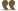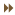# Thread: The Complete Composing Guide

1. woa what? im in 3ml and you cant change tempo for diferent tracks? ooh this might be easier!Reply With Quote

2. D= i still dont knoblehhhhh i just need way to rest at begining without rounding thing happening =(
i think)
can diferent notes be rounded?? will it round if i change note length?Reply With Quote

3. i did it!! i just change tempo in bottom row at same time it changed in top row!!Reply With Quote

4. ##Originally Posted by evantideMC Hammer: Can't Touch This Opening

t120v7o6rrl8raarrbb;
o5rrl8reerrgg;
o5rrl8rccrrdd;
o5>dl8c>barregrr<c>a<cd>a;
o4>dl8c>barregrr<c>a
Volume is at 7.. It should be much higher. AA uses a scale of 0 to 127 for the volume. At 7 you won't hear anything. Please note that the default tempo in AA is 120 so it is not necessary to include it in the code in this example. On the other hand, default volume is 100. I usually set the default volume at 127 for any given track... and work my way down if I have to adjust other tracks. Here's an optimized version(It saves 16 characters total).

Code:
`v127r2l8ro6aar4bbr4.<eer4ggr4.ccr4dd>dc>bar4egr4<c>a<cd>a<<dc>bar4egr4<c>a`Originally Posted by KraylithEvery MML I've looked up so far hasn't been able to play after a simple copy+paste. For example:

Does the original post address this? Do I hafta actually read the whole thing, then go in and make a few edits?
Pretty much the same issue volume is wayyy too low. Here's a the fixed version:
Code:
`MML@v127l64c&c&c&c&c&c&c&c&c&c&c&c&c&c&c&c&c&c&c&c&c&c&c&c&c&c&c&c&c&c&c&c&c&c&c&c&c&c&c&c&c&c&c&c&c&c&c&cl4t75o5l4ddddccccddddccccddddccccddddccccffffcccc<b-b-b-b-aagg>ddddcccc<b-b-b-b-aaa2l16t85o2g>dab->d<b-ag<g>dab->d<b-ag<f>dfa>d<afd<f>dfa>d<afd<g>dab->d<b-ag<g>dab->d<b-ag<da>dfafdfa>de-fafd<f<b->fb->cdc<b-f<b->fb->cdc<b-f<f>cga>c<agf<f>cga>c<agf<e-b->e-fgfe-dcg>cde-dc<g<g>dab->d<b-ab-g4r4<b->dfb->dfb->dfd<b-fd<b-fd<fa>cfa>cfa>c<afc<adc<ab->dfb->dfb->dfd<b-fd<b-fd<fa>cfa>cfa>c<afc<afc<ag>dab->d<b-ag<b->dab->d<b-ag<f>dfa>d<afd<f>dfa>d<afd<e-b->e-fgfe-dcg>cde-dc<g<b->dab->d<b-ab-g4r4l8<b->>ddddddd<<e->>e-e-e-e-e-e-e-<<c>>e-e-e-e-e-e-e-<<f>>fffffff<<d>>ddddddd<<g8>>ddddddd<<c>>ccccccc<<d>b-b-b-b-b-b-b-<d>aaaaaaal16<g>dab->d<b-agdgab->e-<b-ag<g>dab->f<b-agdgab->e-<b-ag;`Originally Posted by EinzbernAlso, has anyone had the issue of 3ML not showing all the tracks? In particular, I wanted to do the Theme of Prontera from Ragnarok Online. This is the midi I downloaded. Whenever I open it up in a normal media player, it plays just fine but when I open it in 3ML it doesn't show all the tracks and the song sounds incomplete. Anyone know what the deal is?
It's a conflict between the MIDI format and MML. In a midi track, multiple notes can occur at the same time as opposed to MML where only one note is possible. To recover those missing note you need to import tracks 1 by one and ensure that you have the 'Convert one MIDI track into multiple MML' checked (See screenshot). Guitar, piano and drum track generally needs to be imported that way since the chances that multiple events occurs at the same time on the same track are pretty high. I would also recommend opening multiple instance of 3MLE, importing your track into different instance of 3MLE and import the single track in another instance where the all the other simple track were imported without using 'Convert one MIDI track into multiple MML' option and just copy paste the content of your guitar & piano tracks into this one.Reply With Quote

5. Bonjour

Est-il possible de passer une commande"Limp Bizkit Mission Impossible Guitar" le lien=> https://www.youtube.com/watch?v=FDwJ_UgfnJc

Merci.

OresteReply With Quote

6. While I cannot understand the language I can understand the title of the song so try this.

o4t100r8l8.d+d+d+d+f+8g+16f+16d+d+d+d+8d+16c+8d8d+ d+d+d+f+8g+16f+16d+d+d+d+8d+16c+8d8d+d+d+d+f+8g+16 f+16d+d+d+d+8d+16c+8d8d+d+d+d+f+8g+16f+16d+d+d+d+8 d+16c+8d8d+d+d+d+f+8g+16f+16d+d+d+d+8d+16c+8d8d+d+ d+d+f+8g+16f+16d+d+d+d+8d+16c+8d8d+d+d+d+f+8g+16f+ 16d+d+d+d+8d+16c+8d8d+d+d+d+f+8g+16f+16d+d+d+d+8d+ 16c+8d8d+d+d+d+f+8g+16f+16d+d+d+d+8d+16c+8d8d+d+d+ d+f+8g+16f+16d+d+d+d+8d+16c+8d8d+d+d+d+f+8g+16f+16 d+d+d+d+8d+16c+8d8d+d+d+d+f+8g+16f+16d+d+d+d+8d+16 c+8d8d+d+d+d+f+8g+16f+16d+d+d+d+8d+16c+8d8d+d+d+d+ f+8g+16f+16d+d+d+d+8d+16c+8d8d+d+d+d+f+8g+16f+16d+ d+d+d+8d+16c+8d8d+d+d+d+f+8g+16f+16d+d+d+d+8d+16c+ 8d8d+d+d+d+f+8g+16f+16d+d+d+d+8d+16c+8d8d+d+d+d+f+ 8g+16f+16d+d+d+d+8d+16c+1,o4l8.d+d+d+d+f+8g+16f+16 d+d+d+d+8d+16c+8d8d+d+d+d+f+8g+16f+16d+d+d+d+8d+16 c+8d8d+d+d+d+f+8g+16f+16d+d+d+d+8d+16c+8d8d+d+d+d+ f+8g+16f+16d+d+d+d+8d+16c+8d8d+d+d+d+f+8g+16f+16d+ d+d+d+8d+16c+8d8d+d+d+d+f+8g+16f+16d+d+d+d+8d+16c+ 8d8d+d+d+d+f+8g+16f+16d+d+d+d+8d+16c+8d8d+d+d+d+f+ 8g+16f+16d+d+d+d+8d+16c+8d8d+d+d+d+f+8g+16f+16d+d+ d+d+8d+16c+8d8d+d+d+d+f+8g+16f+16d+d+d+d+8d+16c+8d 8d+d+d+d+f+8g+16f+16d+d+d+d+8d+16c+8d8d+d+d+d+f+8g +16f+16d+d+d+d+8d+16c+8d8d+d+d+d+f+8g+16f+16d+d+d+ d+8d+16c+8d8d+d+d+d+f+8g+16f+16d+d+d+d+8d+16c+8d8d +d+d+d+f+8g+16f+16d+d+d+d+8d+16c+8d8d+d+d+d+f+8g+1 6f+16d+d+d+d+8d+16c+8d8d+d+d+d+f+8g+16f+16d+d+d+d+ 8d+16c+8d8d+d+d+d+f+8g+16f+16d+d+d+d+8d+16c+8d1,o4 l1rrrr<d+f+c-c+d+f+c-c+l16d+d+d+d+d+d+d+d+d+d+d+d+d+d+d+d+f+f+f+f+f+f+f +f+f+f+f+f+f+f+f+f+<bbbbbbbbbbbbbbbb>c+c+c+c+c+c+c +c+c+c+c+c+c+c+c+c+d+d+d+d+d+d+d+d+d+d+d+d+d+d+d+d +f+f+f+f+f+f+f+f+f+f+f+f+f+f+f+f+<bbbbbbbbbbbbbbbb >c+c+c+c+c+c+c+c+c+c+c+c+c+c+c+c+d+d+d+d+d+d+d+d+d +d+d+d+d+d+d+d+f+f+f+f+f+f+f+f+f+f+f+f+f+f+f+f+<bb bbbbbbbbbbbbbb>c+c+c+c+c+c+c+c+c+c+c+c+c+c+c+c+d+1 f+1c-1c+1d+d+d+d+d+d+d+d+d+d+d+d+d+d+d+d+f+f+f+f+f+f+f+ f+f+f+f+f+f+f+f+f+<bbbbbbbbbbbbbbbb>c+c+c+c+c+c+c+ c+c+c+c+c+c+c+c+c+d+d+d+d+d+d+d+d+d+d+d+d+d+d+d+d+ f+f+f+f+f+f+f+f+f+f+f+f+f+f+f+f+<bbbbbbbbbbbbbbbb> c+c+c+c+c+c+c+c+c+c+c+c+c+c+c+c+d+8.c+l8.d+d+d+1&d +4.d+8r32c+16d+d+d+1,o4l1rrrrr4.<d+f+c-c+d+f+c-c+&c+&c+&c+&c+&c+&c+&c+&c+&c+&c+&c+&c+d+f+c-c+2&c+16.l1.rrrrrr2r32>c+16l8.d+d+d+d+8f+8g+d+d+d+ d+f+8g+r32c+16d+d+d+d+8f+8g+d+d+d+d+f+8g+2,l1.rrrr rrrrrrr1l16o6f+d+<a+2.&a+8>f+d+<a2.&a8>f+d+<a+2.&a +8.ra2&a8a+4>f+d+<a+2.&a+8.ra2.&a8a+2.&a+8>f+d+f+d +<a2l8&ara+l1&a+rrrl16>f+d+<a+2.&a+8>f+d+<a2.&a8>f +d+<a+2.&a+8.ra2&a8a+4>f+d+<a+2.&a+8.ra2.&a8r8a+2. >f+d+f+d+<a2l8&aa+&a+.,o4l1.rrrrrrrrrrrrrrrrrrrrrr rrc+16l8.d+d+d+d+8f+8g+d+d+d+d+f+8g+r32c+16d+d+d+d +8f+8g+d+d+d+d+f+8g+2,o4l1.rrrrrrrrrrrrrrrrrrrrrrr rl8.<c+c+16d+d+d+8f+8g+d+d+d+d+f+8g+r32c+c+16d+d+d +8f+8g+d+d+d+d+f+8g+2,o5l1.rrrrrrrrrrr1l16o6f+d+<a +2.&a+8>f+d+<a2.&a8>f+d+<a+2.&a+8>f+d+<a2.&a8>f+d+ <a+2.&a+8>f+d+<a2.&a8>f+d+<a+2.&a+8>f+d+<a2.&a8>f+ d+<a+2.&a+8>f+d+<a2.&a8>f+d+<a+2.&a+8>f+d+<a2.&a8> f+d+<a+2.&a+8>f+d+<a2.&a8>f+d+<a+2.&a+8>f+d+<a2.&a 8>f+d+<a+2.&a+8>f+d+<a2.&a8>f+d+<a+2.&a+8>f+d+<a2. &a8

Of course this is only part of the song and instrumental only but it gets the idea across. Hope you like itReply With Quote

7. Bonjour Zuesprime

Merci pour ton aide.Reply With Quote